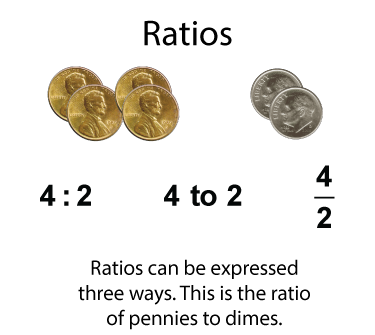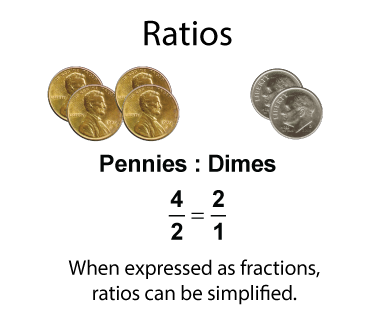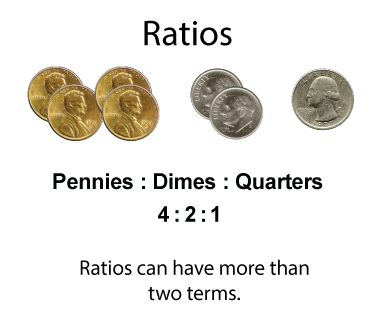# Closed Captioned Video: Ratios: Application of Ratios: Roofs and Ramps

## What Are Ratios?

A ratio is the relationship between two or more quantities among a group of items. Let's look at an example.Here is a collection of coins. The ratio of pennies to dimes can be expressed as a ratio, and a ratio can be written in three different, but equivalent ways.

When expressed as fractions, ratios can then be rewritten as fractions in simplest form.For some groupings, you can write a ratio that includes three terms.Ratios usually include similar types of objects. Here is a collection of different types of balls.Rates are a special type of ratio. Rates involve different types of quantities. Here is an example of a rate involving cost and weight.In this video, we explore the application of ratio concepts to the pitch of a roof and the slope of a ramp. Several real-world examples are explored.

## Video Transcripts

### This video has a transcript available. To see the complete collection of video transcripts, click on this link.

Common Core Standards CCSS.MATH.CONTENT.6.RP.A.3, CCSS.MATH.CONTENT.7.RP.A.2, CCSS.MATH.CONTENT.6.RP.A.1, CCSS.MATH.CONTENT.7.RP.A.1 3.67 minutes 6 - 8 Algebra     • Ratios, Proportions, and Percents         • Ratios and Rates 2020 percents, percents video tutorials, pitch, slope, roof, video tutorial, Closed Captioned Video, ramp